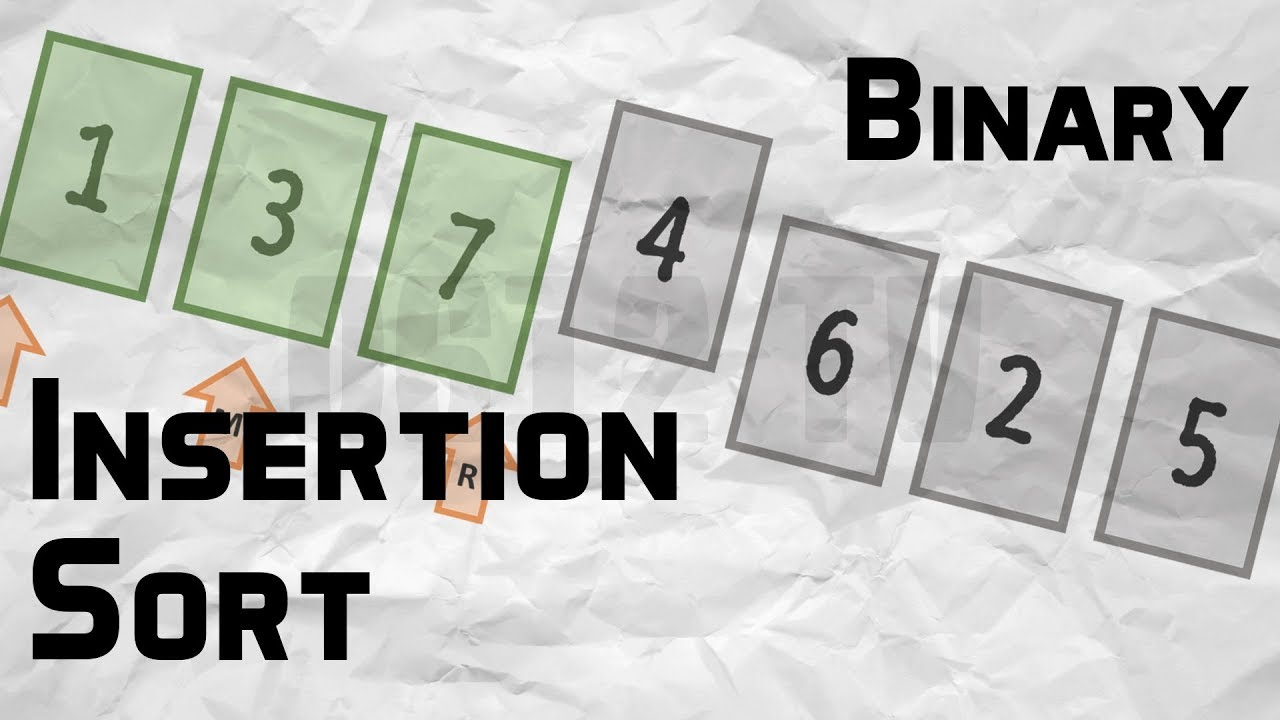# When does the worst case of Quicksort occur?

When does the worst case of Quicksort occur? – Searching and sorting – Since these cases are very common use cases, the problem was easily solved by choosing either a random index for the pivot.

The answer depends on strategy for choosing pivot. In early versions of Quick Sort where leftmost (or rightmost) element is chosen as pivot, the worst occurs in following cases.

1) Array is already sorted in same order.
2) Array is already sorted in reverse order.
3) All elements are same (special case of case 1 and 2)

Since these cases are very common use cases, the problem was easily solved by choosing either a random index for the pivot, choosing the middle index of the partition or (especially for longer partitions) choosing the median of the first, middle and last element of the partition for the pivot. With these modifications, the worst case of Quick sort has less chances to occur, but worst case can still occur if the input array is such that the maximum (or minimum) element is always chosen as pivot.

##### You May Also Like## Binary Insertion Sort

Binary Insertion Sort – Searching and Sorting – We can use binary search to reduce the number of comparisons in normal insertion sort. Binary Insertion Sort find use binary search to find the proper location to insert the selected item at each iteration.

## Add 1 to a given number

Add 1 to a given number – Bit Algorithm – Add 1 to a given number write a program to add 1 to a given number. You are not allowed to use operators like ‘+’,